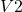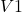## If a moving clock is ticking half as fast as normal what speed is the clock traveling?

Question

If a moving clock is ticking half as fast as normal what speed is the clock traveling?

in progress 0
2 months 2021-07-25T17:09:16+00:00 1 Answers 5 views 0

Speed of moving clock is=πr/(86400) m/s

Here,

m and s are SI units of distance and time respectively.

Explanation:

If

than,

total distance covered on clock=S=2πr m

here, m=SI unit of distance

and required time for covering the total distance=t= 86400s

speed of normal clock==S/t=2πr/86400 m/s

As, moving moving clock is ticking half as fast as normal clock so,

speed of moving clock==/2=2πr/(86400)*2 m/s=πr/(86400) m/s